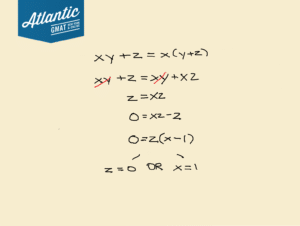# If xy + z = x(y + z), which of the following must be true? GMAT Explanation + Additional Examples!

If xy + z = x(y + z), which of the following must be true?

1. x = 0 and y = 0
2. x = 1 and y = 1
3. y = 1 and z = 0
4. x = 1 or y = 0
5. x = 1 or z = 0

## Define the question:

There are two things that are important to note.

1. Which of the following must be true? The keyword here is “must.” The question is not asking which of these can be true – so we aren’t just plugging in answer choices and seeing if the equation works out. We need to do some work to deduce what must be true about the equation.
2. The answer choices have slight differences. A, B, and C are all “and” statements, while D and E are “or” statements.

So, for answers A, B, or C to be correct, we’ll need both variables to have their specified value, and for D and E, either variable having its specified value on its own will suffice.

## Algebra time:

Let’s dig into: xy + z = x(y + z).

Our first step is to simplify by distributing out the ‘x’ on the right-side.

xy + z = xy + xz.

We now have an ‘xy’ term on either side. This works out quite nicely, as we can subtract xy from both sides, making the equation much simpler:

z = xz.

Let’s get everything to one side and factor:

0 = xz – z

0 = z(x – 1)

From here, we can see that this equation is satisfied by either z = 0 or x = 1.

Here is all of that worked out:

#Alternate Solution:

Going back a couple steps, let’s think more about what subtracting ‘xy’ from both sides actually means. When we end up at the simplified equation:

z = xz

There’s no ‘y’ to be found anywhere.

Since we were able to subtract out ‘y’ from the equation, we are essentially saying that the value of y does not matter. In other words: y could be anything.

The question specified that the values of the variables must be true

As the value of y could be anything, we can effectively rule out any answer choice that specifies a value for y.

That rules out everything but E.